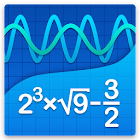Graphing Calculator + Math, Algebra & Calculus

• 검색 유형

모든 Android 애플리케이션 카테고리

모든 Android 게임 카테고리# Graphing Calculator + Math, Algebra & Calculus

78K 9

9 사용자
평가 등급

## 스크린샷

설명

Graphing Calculator by Mathlab is a scientific graphing calculator integrated with algebra and is an indispensable mathematical tool for students from high school to those in college or graduate school, or just anyone who needs more than what a basic calculator offers. It is designed to replace bulky and costly handheld graphing calculators and works on virtually any Android phone or tablet.

Video: https://youtu.be/6BR8Lv1U9kA
Help site with instructions and examples: http://help.mathlab.us
If you have a question, send email to calc@mathlab.us

SCIENTIFIC CALCULATOR
• Square root, cube and higher roots (hold √ key)
• Exponent or power, use x^ key, (x^2)
• Logarithms ln(), log(), log[base]()
• Trigonometric functions sin π/2, cos 30°, ...
• Hyperbolic functions sinh, cosh, tanh, ... (hold "e" key to switch)
• Inverse functions (hold direct function key)
• Complex numbers, all functions support complex arguments
• Derivatives sin x' = cos x, ... (hold x^ key)
• Scientific and engineering notation (enable in menu)
• Percent mode
• Binary, octal and hexadecimal numbers, 0b1010, 0o123, 0xABC

GRAPHING CALCULATOR
• Multiple functions graphing
• Implicit functions up to 2nd degree (ellipse 2x^2+3y^2=1, etc.)
• Polar graphs (r=cos2θ)
• Parametric functions, enter each on new line (x=cos t, y=sin t)
• Function roots and critical points.
• Graph intersections
• Tracing function values and slopes
• Slide to scroll
• Pinch to zoom
• Fullscreen graphs (PRO)
• Function tables
• Save graphs as images
• Save tables as csv
• 3D graphs (PRO)

FRACTION CALCULATOR
• Simple and complex fractions 1/2 + 1/3 = 5/6
• Mixed numbers, use space to enter values 3 1/2
• Use parentheses (1+2)/(3+4)=3/7

ALGEBRA CALCULATOR
• Linear equations x+1=2 -> x=1
• Quadratic equations x^2-1=0 -> x=-1,1
• Approximate roots of higher polynomials
• Systems of linear equations, write one equation per line, x1+x2=1, x1-x2=2
• Polynomial long division
• Polynomial expansion, factoring

PROBABILITY AND STATISTICS
• Hold n! key to enter functions, double tap dot to enter comma
• Combinations nCr(5,2)=10
• Permutations nPr(5,2)=20
• Sample A=[1 2 3 4 5]
• Sample B=[2 3 4 5 6]
• Sum sum(A) = 15
• Average avg(A) = 3
• Median median(A) = 3
• Sample variance var(A) = 2.5
• Population variance varp(A) = 2
• Standard deviation stdev(A) = 1.58..., stdevp(A) = 1.41...
• Covariance cov(A,B) = 2.5
• Correlation corr(A,B) = 1

MATRIX CALCULATOR
• Matrix and vector arithmetic operations
• Vector cross product, dot product (hold *) and norm
• Matrix determinant, inverse, transpose and trace functions

LIBRARY
• Physical constants (PRO)
• User defined constants and functions (PRO)
• Save expressions for future reference

: 78.019 리뷰

"대단함"

9